# Non parametric presentation

22. Jul 2017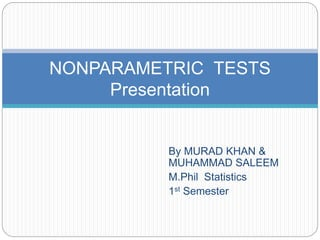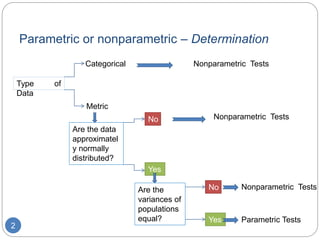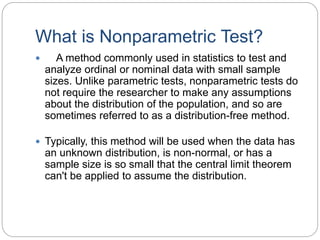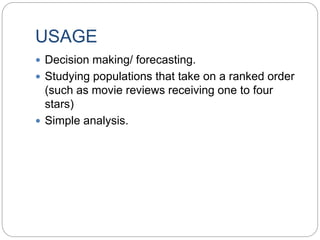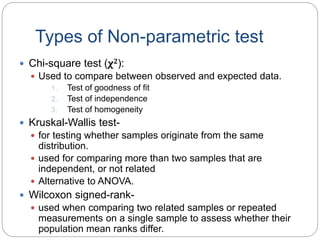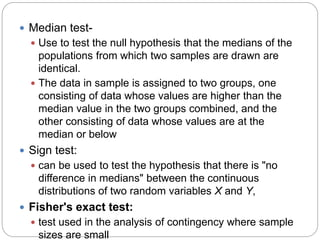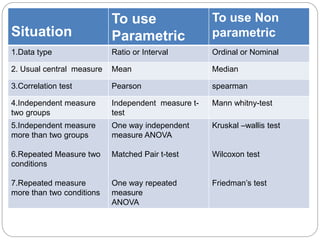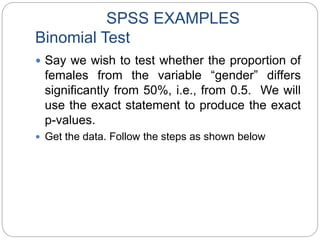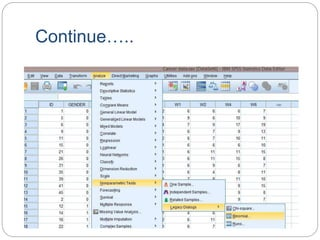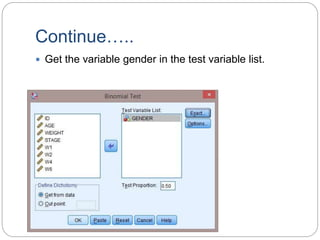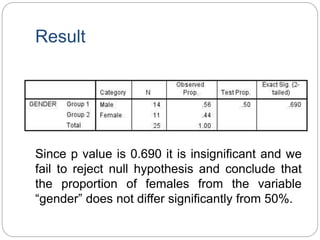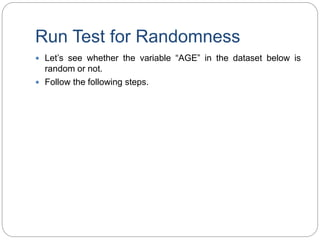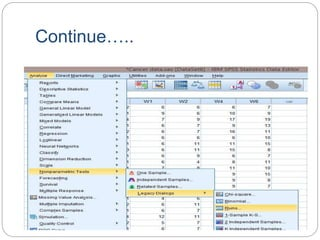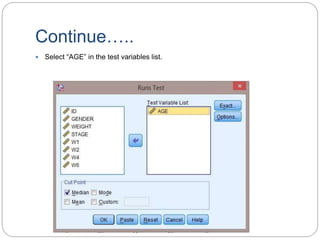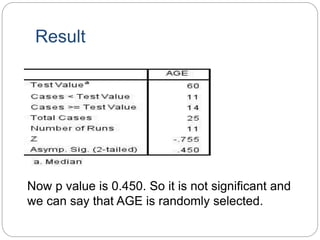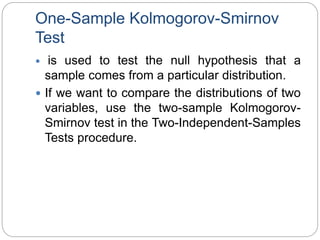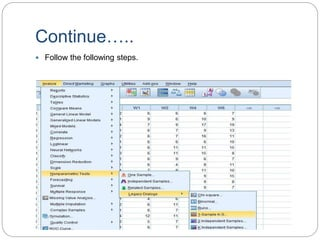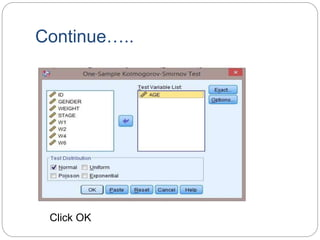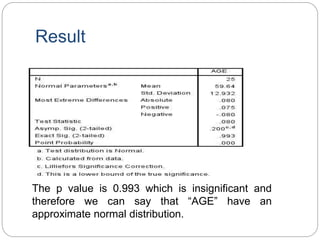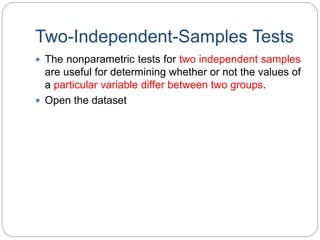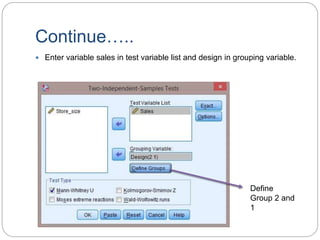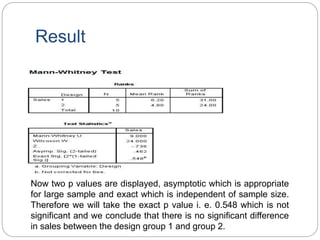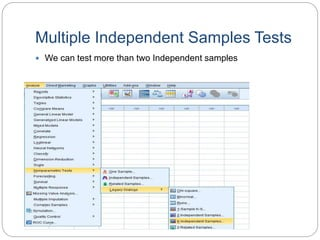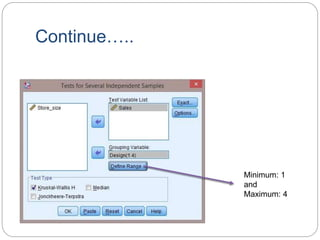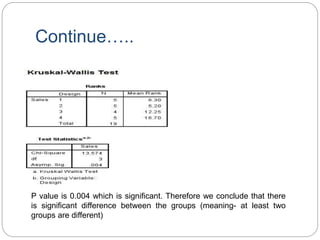1 von 27

### Non parametric presentation

• 1. By MURAD KHAN & MUHAMMAD SALEEM M.Phil Statistics 1st Semester Department of statistics Abdul Wali Khan Unicersity Mardan KPK NONPARAMETRIC TESTS Presentation
• 2. Parametric or nonparametric – Determination 2 Type of Data Categorical Metric Are the data approximatel y normally distributed? Yes No Are the variances of populations equal? Nonparametric Tests Parametric Tests Nonparametric Tests No Nonparametric Tests Yes
• 3. What is Nonparametric Test?  A method commonly used in statistics to test and analyze ordinal or nominal data with small sample sizes. Unlike parametric tests, nonparametric tests do not require the researcher to make any assumptions about the distribution of the population, and so are sometimes referred to as a distribution-free method.  Typically, this method will be used when the data has an unknown distribution, is non-normal, or has a sample size is so small that the central limit theorem can't be applied to assume the distribution.
• 4. USAGE  Decision making/ forecasting.  Studying populations that take on a ranked order (such as movie reviews receiving one to four stars)  Simple analysis.
• 5. Types of Non-parametric test  Chi-square test (χ2):  Used to compare between observed and expected data. 1. Test of goodness of fit 2. Test of independence 3. Test of homogeneity  Kruskal-Wallis test-  for testing whether samples originate from the same distribution.  used for comparing more than two samples that are independent, or not related  Alternative to ANOVA.  Wilcoxon signed-rank-  used when comparing two related samples or repeated measurements on a single sample to assess whether their population mean ranks differ.
• 6.  Median test-  Use to test the null hypothesis that the medians of the populations from which two samples are drawn are identical.  The data in sample is assigned to two groups, one consisting of data whose values are higher than the median value in the two groups combined, and the other consisting of data whose values are at the median or below  Sign test:  can be used to test the hypothesis that there is "no difference in medians" between the continuous distributions of two random variables X and Y,  Fisher's exact test:  test used in the analysis of contingency where sample sizes are small
• 7. Situation To use Parametric To use Non parametric 1.Data type Ratio or Interval Ordinal or Nominal 2. Usual central measure Mean Median 3.Correlation test Pearson spearman 4.Independent measure two groups Independent measure t- test Mann whitny-test 5.Independent measure more than two groups 6.Repeated Measure two conditions 7.Repeated measure more than two conditions One way independent measure ANOVA Matched Pair t-test One way repeated measure ANOVA Kruskal –wallis test Wilcoxon test Friedman’s test
• 8. SPSS EXAMPLES Binomial Test  Say we wish to test whether the proportion of females from the variable “gender” differs significantly from 50%, i.e., from 0.5. We will use the exact statement to produce the exact p-values.  Get the data. Follow the steps as shown below
• 9. Continue…..
• 10. Continue…..  Get the variable gender in the test variable list.
• 11. Result Since p value is 0.690 it is insignificant and we fail to reject null hypothesis and conclude that the proportion of females from the variable “gender” does not differ significantly from 50%.
• 12. Run Test for Randomness  Let’s see whether the variable “AGE” in the dataset below is random or not.  Follow the following steps.
• 13. Continue…..
• 14. Continue…..  Select “AGE” in the test variables list.
• 15. Result Now p value is 0.450. So it is not significant and we can say that AGE is randomly selected.
• 16. One-Sample Kolmogorov-Smirnov Test  is used to test the null hypothesis that a sample comes from a particular distribution.  If we want to compare the distributions of two variables, use the two-sample Kolmogorov- Smirnov test in the Two-Independent-Samples Tests procedure.
• 17. Continue…..  Follow the following steps.
• 18. Continue….. Click OK
• 19. Result The p value is 0.993 which is insignificant and therefore we can say that “AGE” have an approximate normal distribution.
• 20. Two-Independent-Samples Tests  The nonparametric tests for two independent samples are useful for determining whether or not the values of a particular variable differ between two groups.  Open the dataset
• 21. Continue…..  Let’s compare between design 1 and 2.
• 22. Continue…..  Enter variable sales in test variable list and design in grouping variable. Define Group 2 and 1
• 23. Result Now two p values are displayed, asymptotic which is appropriate for large sample and exact which is independent of sample size. Therefore we will take the exact p value i. e. 0.548 which is not significant and we conclude that there is no significant difference in sales between the design group 1 and group 2.
• 24. Multiple Independent Samples Tests  We can test more than two Independent samples
• 25. Continue….. Minimum: 1 and Maximum: 4
• 26. Continue….. P value is 0.004 which is significant. Therefore we conclude that there is significant difference between the groups (meaning- at least two groups are different)